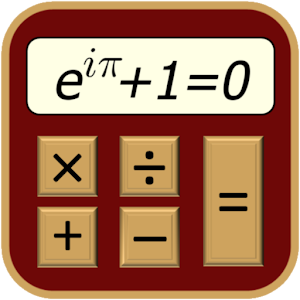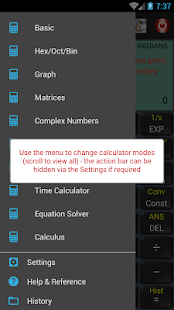Scientific Calculator (adfree) 4.0.5 APK for AndroidModes included are:

• Basic Mode,
• Scientific Mode,
• 64-bit Programmer Mode (Hex, Oct, Bin and Dec),
• Graphing (save copy of graph to sdcard),
• Matrices,
• Complex Numbers (cartesian, polar, using Euler’s identity),
• Quick Formulas,
• Quick Converter,
• Time Calculator,
• Equation Solver,
• Calculus (Derivative, Definite Integral, Taylor Series & Indefinite Integral) – requires Android version 2.3 or higher
• Financial
+ the Periodic Table of Elements!
Features include:
• All Trigonometric operations (radians, degrees or gradients)
• Powers & Roots
• Logs and Antilogs
• Factorial, Modulus & Random Numbers functions
• HCF, LCM, Prime factorization
• Pol() & Rec() Functions
• Permutations (nPr) and Combinations (nCr)
• Statistics
• Fractions Mode
• A wide range of conversion categories and constants
• 20 Memory Registers in each of the calculation modes
• Detailed calculation history
• Extensive Help and Reference
• Highly customizable via the Settings
The reference section includes:
• Physical Laws
• Names in the Metric System
• Mathematical Tables
• Elementary & Linear Algebra
• Trigonometric Identities
• Differentiation & Integration Rules
• Statistics Formulas
• Vector Mathematics
• ASCII, Fractional Bits & Roman Numeral Converters
• pH, Interpolation, Body Mass Index (BMI), Percentage & Proportion Calculators
• Sigma & Pi Notation
Please email any questions that are not answered in the Help section.

WHAT’S NEW:

13/02 (ver 4.0.5)
* added Humidity Calculations to the Reference Section